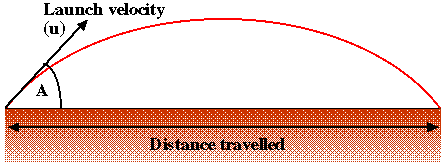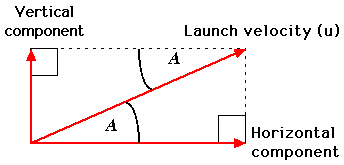# (Week 6 Starting Monday October 30 2006)

### Hand in date - one week after your scheduled tutorial session in week 6.

Aims and Objectives: Provide an opportunity for students to design and implement a more substantial software system (than those produced for parcticals 1 and 2) that, in addition, requires use of methods contained in the Math class found in the Java API.

## 1. REQUIREMENTS

Produce a computer program, written in the Java programming language, which determines the distance travelled by a projectile (projected from the ground) given:

1. Velocity at launch (u), and
2. Launch angle (angle of elevation) above the horizontal (A).Assume the following:

• The angle of elevation is given in degrees and is in the range of 0 to 90.
• Start velocity is given as a positive number.
• Acceleration due to gravity (g) is equivalent to 10m/s^2.
• The effect of air resistance can be ignored.

Note also that to solve the above we must carry out the following steps.

1. Calculate the vertical and horizontal components of u (the launch velocity) using the following trigonometric identities:
```Vertical component of launch velocity  (Vu) = u x sinA

Horizontal component of launch velocity (Hu) = u x cosA
```2. Calculate the time (t) taken for the body to return to the ground using the identity:
```t = (2 x Vu)/a
```
where a (deceleration due to gravity) is equivalent to g (10m/s^2 in this case).
3. Calculate distance (s) traveled from the identity:
```s = Hu x t
```

## 2. EXAMPLE

A body is projected with a velocity of u = 200 m/s at an angle of elevation A = 30 degrees above the horizontal. Determine the distance traveled by the projectile.

```Vu  = 200 x sin30 = 100 (m/s)

Hu = 200 x cos30 = 173.2 (m/s)

t = {2 x 100)/10 = 20 (s)

s = 20 x 173.2051 = 3464.1 (m)
```

## 3. REPORT

You should prepare a report comprising the following sections:

1. Requirements: Summary of the above requirements.
2. Analysis and Design: A short (one paragraph) description of your analysis of the problem including a Class Diagram outlining the class structure for your proposed solution and a set of "summary tables". Detailed designs for the methods you intend to include supported by a Nassi-Shneiderman chart for each.
3. Implementation: Your Java source files.
4. Testing: A set of appropriate arithmetic test cases (presented in tabular form) and the output from your program as a result of running these test cases.

All supporting documentation (i.e. excluding your Java source source files) should be prepared as a single Microsoft Word file. Once completed you should "up load" your Java source files (extension .java) and your word document to the CS department's electronic "practical assignment submission" system.

Refer to guidance notes on the execution of COMP101 practicals and the presentation of work if necessary. You might also like to refer to the vertical motion example studied in week 5, and the work on trigonometric ratios studied in week 4.

## 4. MARK SCHEME

90% of the marks will be distributed evenly between: Analysis and Design; Implementation; and Testing. The remaining 10% will be awarded for "overall presentation".